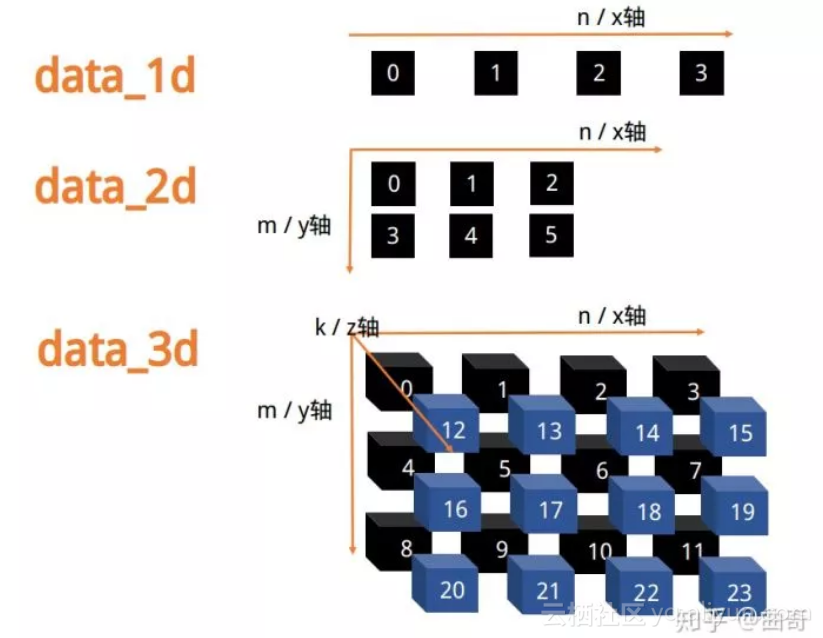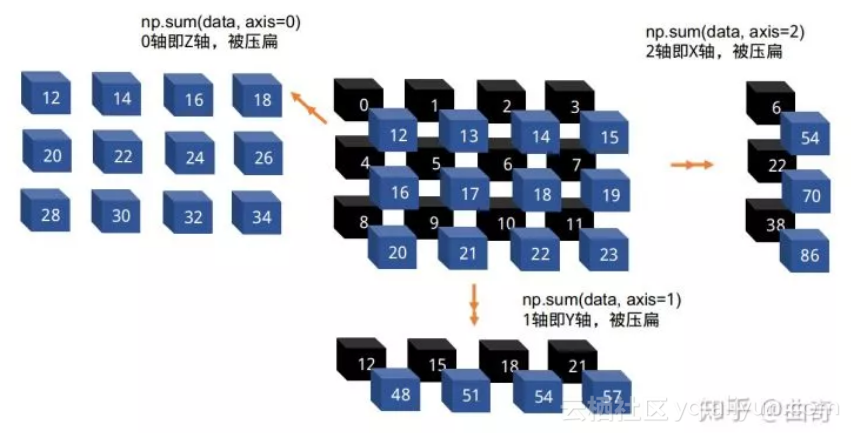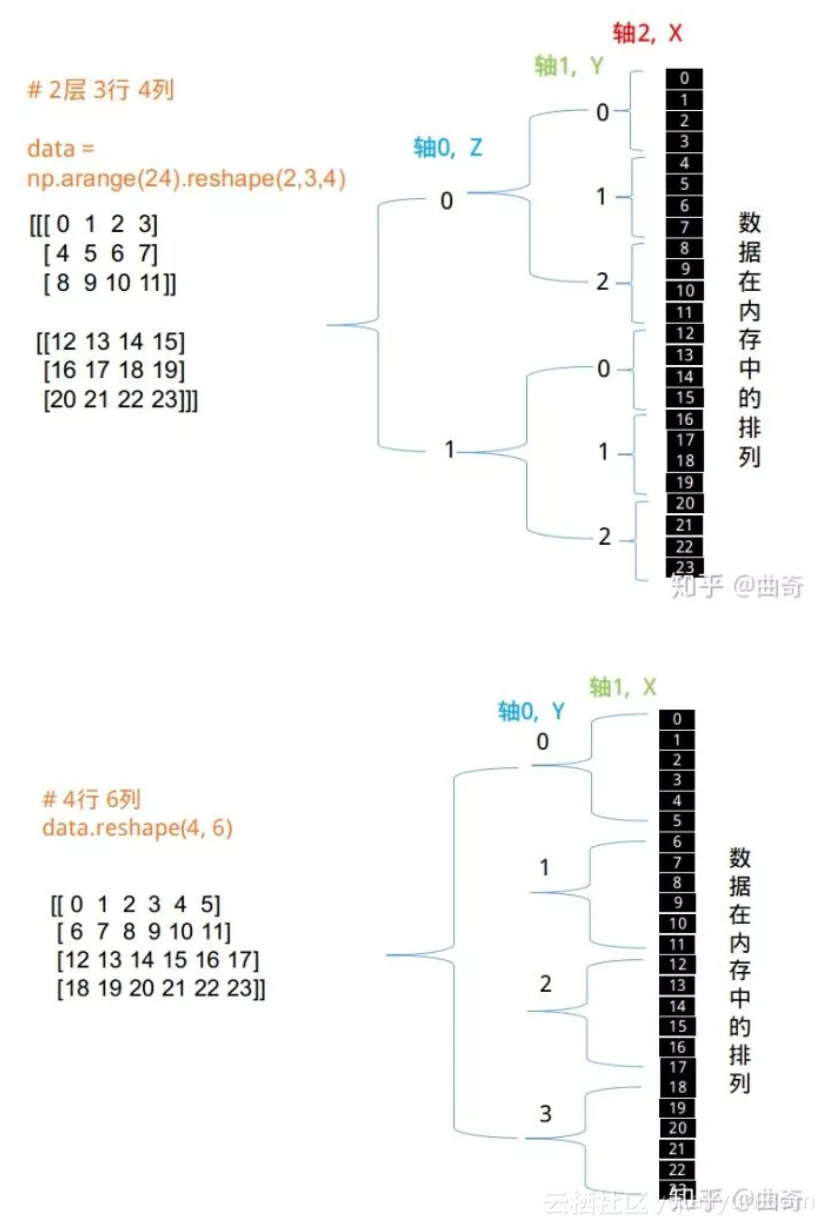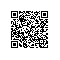# 基础 | numpy ndarray 之内功心法，理解高维操作！

import numpy as np

# 一维数据不用赘言
data_1d = np.array([0, 1, 2, 3])

# 二维数据作为 m 行 n 列的表格，例如 2 行 3 列
data_2d = np.arange(6).reshape(2, 3)

# 三维数据作为 k 层 m 行 n 列 的积木块， 例如 2 层 3 行 4 列
data_3d = np.arange(24).reshape(2, 3, 4)>>> print(data_3d.ndim)
3
>>> print(data_3d.shape)
(2, 3, 4)

shape 是一个很关键的属性，我是这样把它和各个轴对应的：

shape: (2, 3, 4)
k, m, n
z, y, x

import numpy as np
import matplotlib.pylab as plt

image = plt.imread("lena.jpg")
print(image.shape)

# --- 结果 ---
# (480, 640, 3)
# (y, x, c)

>>> print(data.shape)
(3, 3, 2, 5)

# axis 0: 3
# axis 1: 3
# axis 2: 2
# axis 3: 5

data = np.array(np.arange(12))
np.random.shuffle(data)
data = data.reshape(3, 4)
print(data)

# [[10 8 3 2]
# [ 5 6 0 7]
# [11 4 9 1]]

print(np.sort(data, axis=0))

# [[ 5 4 0 1] | 小
# [10 6 3 2] | 到
# [11 8 9 7]] | 大

print(np.sort(data, axis=1))

# 小 到 大
# --------->
# [[ 2 3 8 10]
# [ 0 5 6 7]
# [ 1 4 9 11]]

shape: (3, 4)
axis: 0, 1
AXIS: y, x

2. 求和、均值、方差、最大、最小、累加、累乘

sum，mean，std，var，min，max 会导致这个轴被压扁，缩减为一个数值

data = np.arange(24).reshape(2, 3, 4)
print(data)

# [[[ 0 1 2 3]
# [ 4 5 6 7]
# [ 8 9 10 11]]
#
# [[12 13 14 15]
# [16 17 18 19]
# [20 21 22 23]]]

print( np.sum(data, axis=0) )

# 0轴被sum压扁，1轴2轴不变
# [[12 14 16 18]
# [20 22 24 26]
# [28 30 32 34]]

print( np.sum(data, axis=1) )

# 1轴被sum压扁，0轴2轴不变
# [[12 15 18 21]
# [48 51 54 57]]cumsum，cumprod 不缩减轴向，只在指定轴向操作，请读者自己试验。

3. 索引和切片（indexing and slicing）

data[1:2, 0:1, 0:1] 中，三个轴都保留，但只有一个数据元素，很神奇吧。

data = np.arange(24).reshape(2, 3, 4)
print( data )

# [[[ 0 1 2 3]
# [ 4 5 6 7]
# [ 8 9 10 11]]
#
# [[12 13 14 15]
# [16 17 18 19]
# [20 21 22 23]]]

print( data[0, :, :] )

# axis 0，即 z 轴，是数值，则 z 轴消失，切了一片 x-y
# [[ 0 1 2 3]
# [ 4 5 6 7]
# [ 8 9 10 11]]

print( data[0, 1, 2] )

# 所有轴都消失，只返回一个标量数据
# 6

print( data[0:1, 1:2, 2:3] )

# 返回三维数据，虽然只有一个元素
# [[]]

4. 拼接（concatenating）

data = np.arange(4).reshape(2, 2)
print( np.concatenate([data, data], axis=0) )

# 在轴向 0 拼接，即 y 方向
# [[0 1]
# [2 3]
# [0 1]
# [2 3]]

print( np.concatenate([data, data], axis=1) )

# 在轴向 1 拼接，即 x 方向
# [[0 1 0 1]
# [2 3 2 3]]

reshape 之迷乱int data;
int data;使用钉钉扫一扫加入圈子
+ 订阅